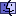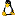Making an Image Translucent Using AlphaDataThis assumes you have a good understanding of how to manipulate `imageData` and `maskData`. If you don’t, take a look at tip imag003 - Understanding ImageData, MaskData and AlphaData before continuing.

The following is an example of making an image 50% transparent using the `alphaData` property of an image. It is similar to setting the `ink` of an image to `blend` and then setting the `blendLevel`, but is useful to show how to manipulate the `alphaData` of an image.

```on mouseUp
put the imageData of image 1 into iData
put the width of image 1 into tW
put the height of image 1 into tH

repeat with i = 1 to tH  -- iterate each row
repeat with j = 1 to tW   -- iterate each column
-- Set every pixel to be 50% transparent (1/2 of 255 = 128)
end repeat
end repeat

set the imageData of image 1 to iData
end mouseUp```
This can be shortened further because you’re using every pixel of the image, so you don’t need to know the height and width of the image. Here’s the shorter version (thanks, Sarah!):

```on mouseUp
put the imageData of image 1 into iData

repeat for each char X in the alphaData of image 1  -- See Note below
-- Set every pixel to be 50% transparent (1/2 of 255 = 128)
 Note: Using this approach provides a way to iterate over the pixels using a `repeat for each` looping construct (which is much faster than a `repeat with` loop). Both `the maskData of image` and `the alphaData of image` will return a number of characters (a "length") that is the total number of pixels in the image (for a refresher on this, see imag003 - Understanding ImageData, MaskData and AlphaData.) So, since I could use either `the maskData of image` or ``` the alphaData of image```, I decided to use `the alphaData of image` to avoid confusion. Hope that’s clear...